|

# 金华十大设计师王乐乐:与心爱的人一起享受诗和远方

生活在快节奏的喧闹都市，我们有多久不曾闻到草木的馨香？又有多久不曾赴大自然的一场约会？曾经充满温情的家，仿佛在不知不觉中失去了浪漫的色彩。

于是，设计师王乐乐把五彩花园搬进家里，让业主重温和爱人在国外度假时的轻松与愉悦；让业主不论何时，只要推开房门漫步在自家花园里，便能将好春光尽收眼底，尽享花园美景，在这里静静的与心爱的人一起享受“诗和远方”般的惬意生活。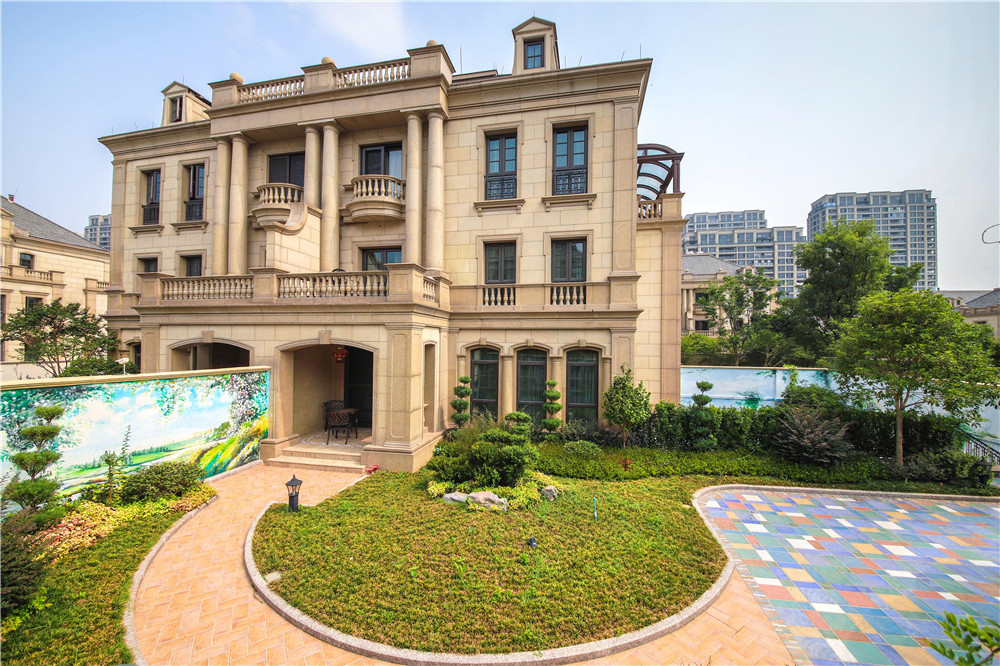项目名称：海棠花园别墅

项目地点：浙江金华

建筑面积：1000平方

完成时间：2017.9

设计师：王乐乐

五彩花园Colorful garden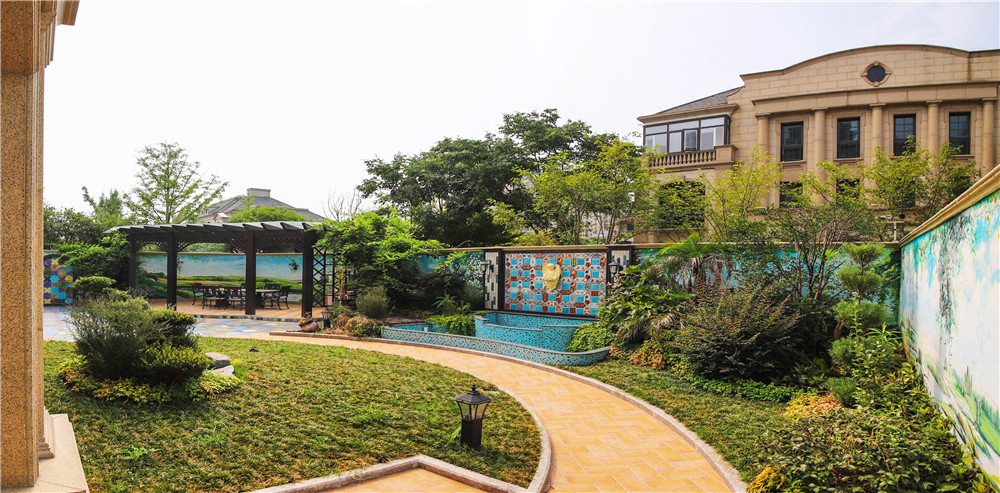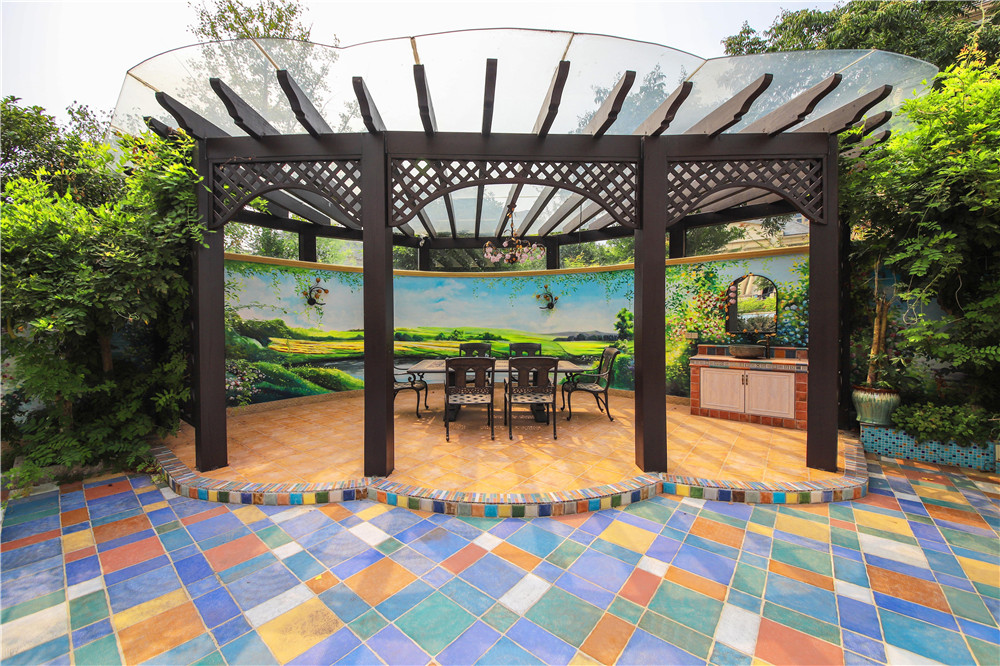芳草连天碧，春色入院来。房子虽然有1000平方米，户外五彩花园却是设计师王乐乐为业主重点打造的一个区域。王老师特意选用了艺术手工仿古砖来营造国外度假休闲的花园场景，弧形的景观水池配上马赛克铺贴和手绘风景画，更是别有一番风味，深入的表达了花园的逸静。不论是春日品花香浓情，还是夏日听雨打芭蕉，抑或秋日看云卷云舒，在这样的花园里，时间好像停住了脚步，让业主尽情享受这里的静好岁月。

玄关Homeentrance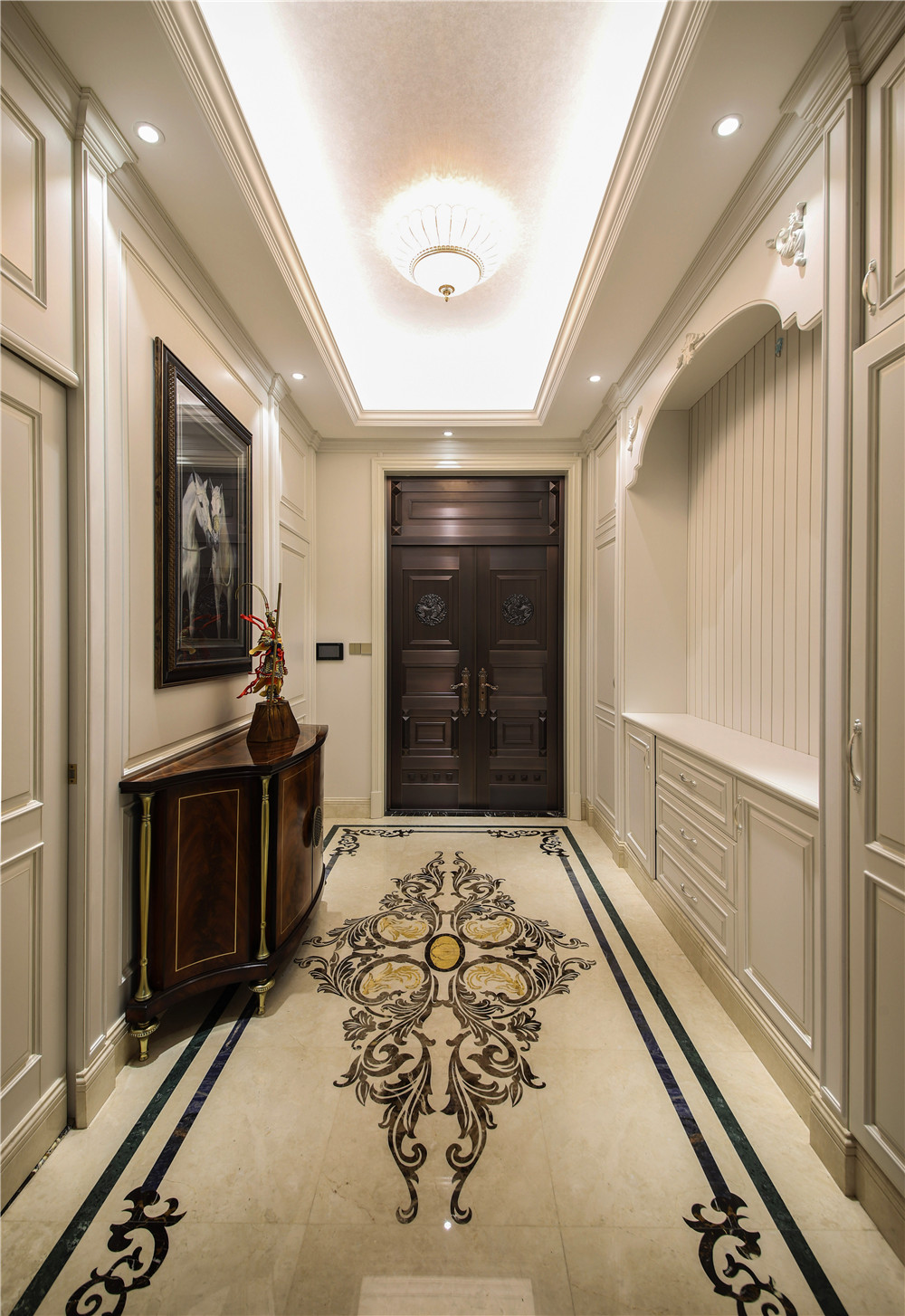进入大门，便是玄关，玄关的设计简单、高贵、富有仪式感，地面通体的花纹大理石搭配富有动感的流线型玄关柜加上护墙板凹凸造型的勾勒，都在诉说着房屋主人是一个年轻、时尚、有个性的人。设计师王乐乐还暖心的设计了储物空间方便放置各类衣帽和鞋子。

客厅Sitting room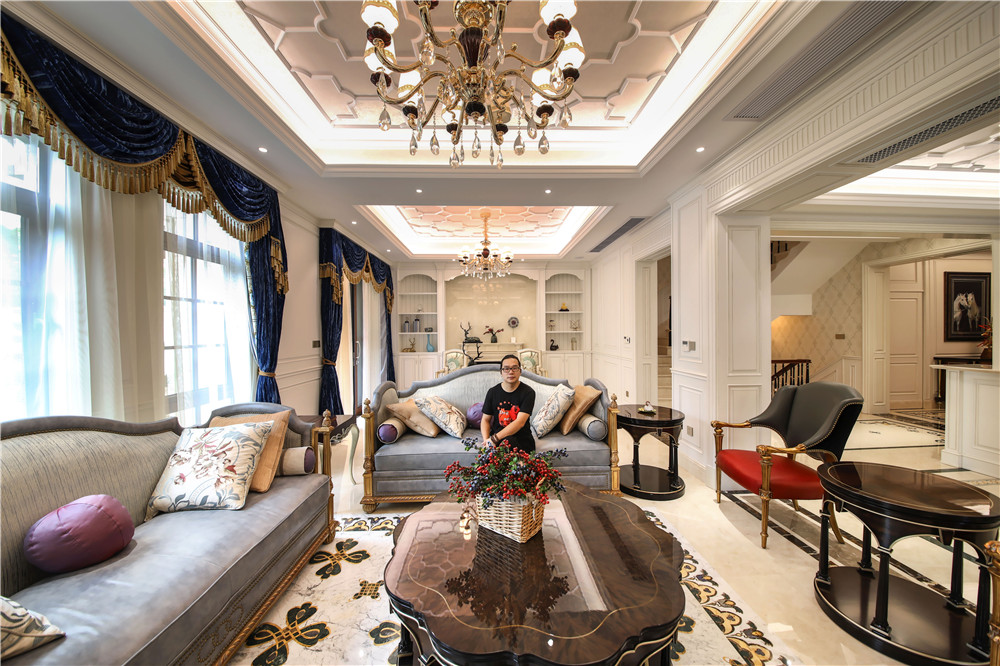一层的客厅拥有一整面墙的落地窗和玻璃门，阳光透过纱帘洒进屋里，留下一地金黄和满屋温暖。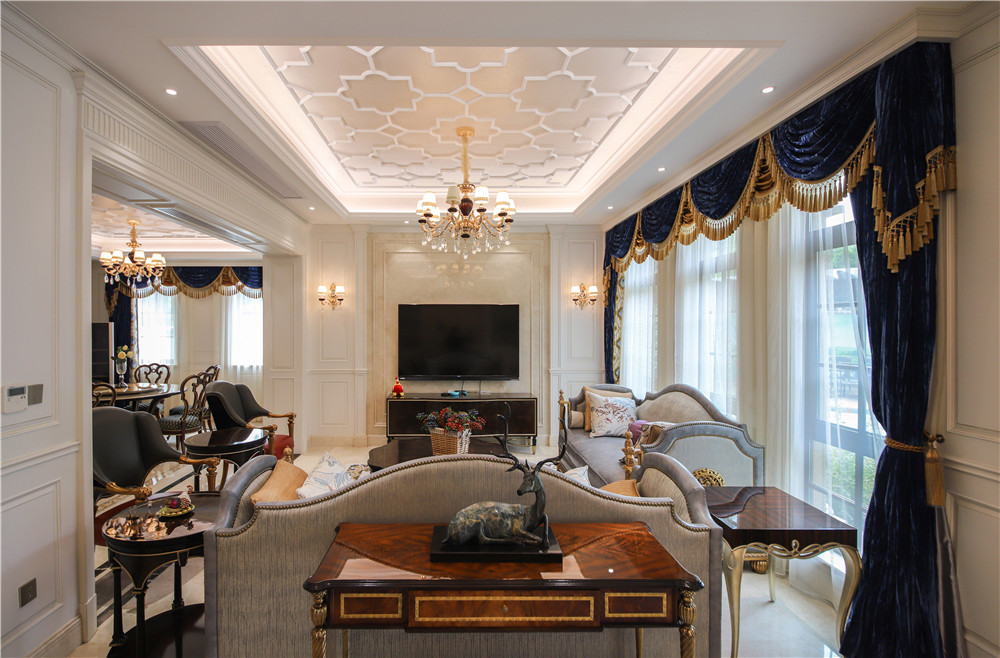在客厅里，设计师王乐乐选择了富贵典雅的皇室蓝色窗帘和干净大气的冰蓝色绒面的拉卡萨真皮沙发，同时搭配金色点缀，提升了空间的层次感。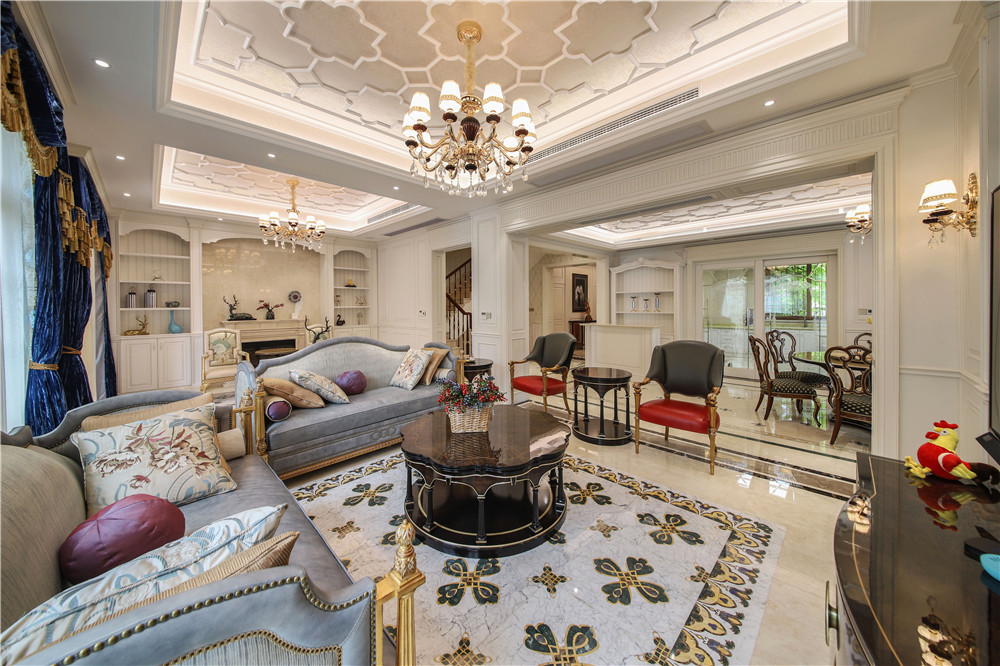而富有质感的黑色大理石桌几，搭配米色大花地毯，更是给人以尊贵的舒适感受。总体而言，客厅的空间虽然大，但是被设计师布置的温馨而充盈，既不杂乱亦不空旷。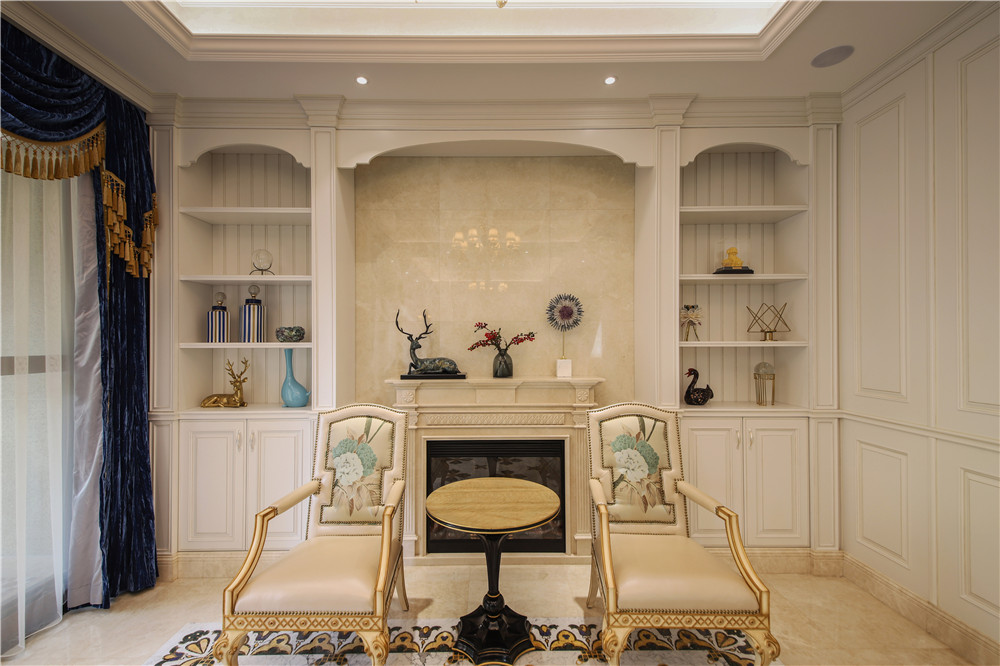位于客厅沙发后侧的壁炉休闲区，是设计师王乐乐特意给业主设计的，可以让喜爱欧美文化的业主时刻联想到一家人在国外居住时其乐融融的场景。壁炉不仅是功能区域，更是一家温暖的集合地，链接家人心灵的纽带。

餐厅Dining room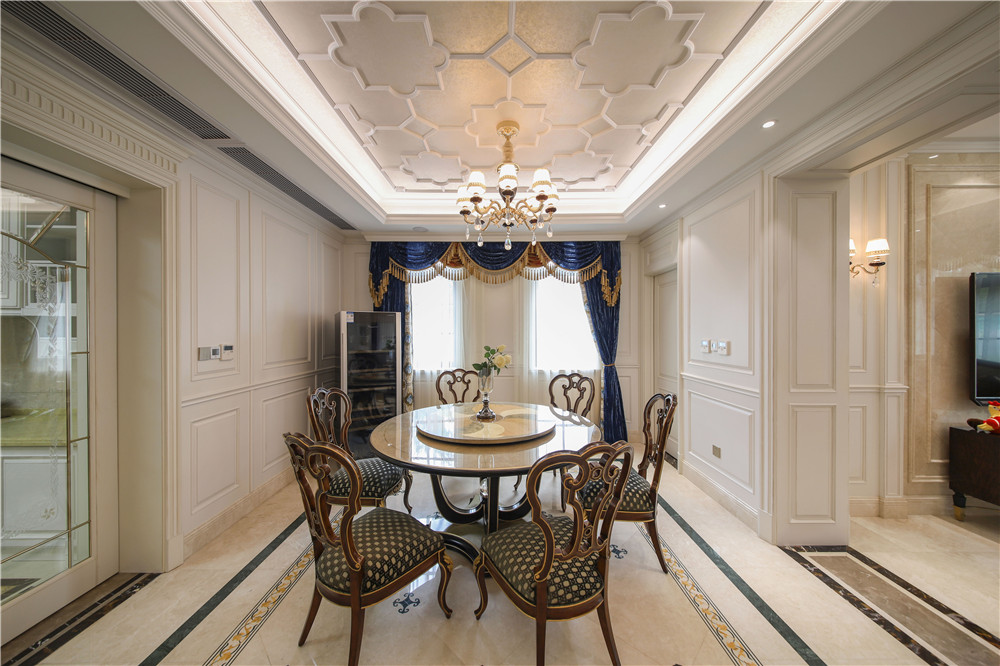餐厅在客厅和厨房的中间，合理的空间布局，让业主用餐十分便利。选用中国人习以为常的纯木色实木圆桌，搭配美式的布面座椅，没有过多繁复的装饰，以实用为主。符合业主对家的态度。

公共卫生间Public toilets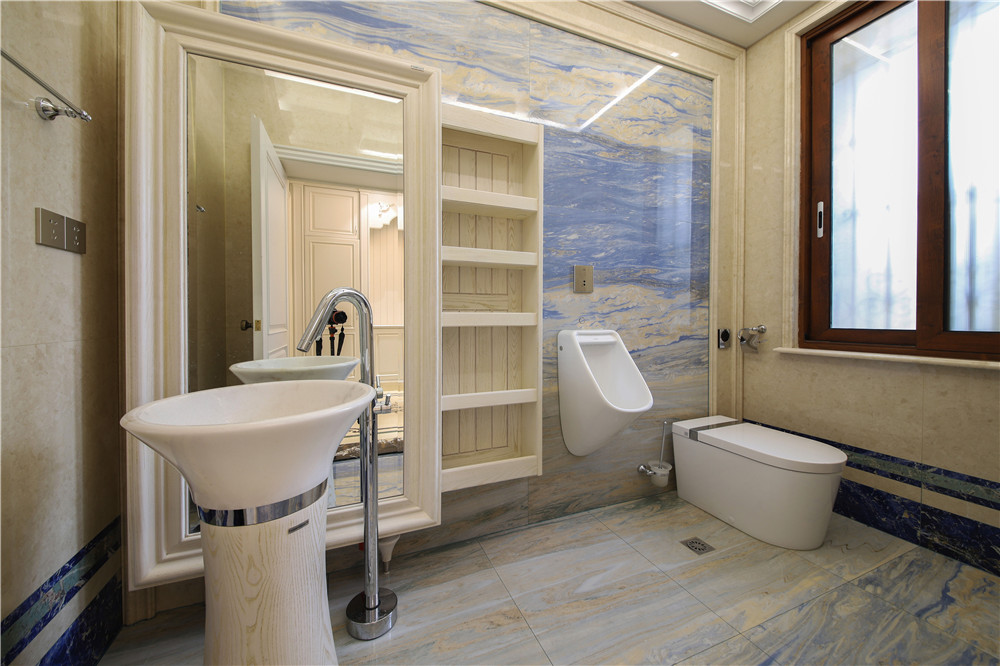位于一楼玄关处有一个公共卫生间，它的门恰好隐藏在玄关处的护墙板中。进入卫生间首先感觉到的是豁亮、时尚。考虑是接待家中客人的，所以仿照的是公共商业空间所设计，特意设有小便池和马桶，以及偌大的镜子和丰富的储物空间，简单实用，让到家的客人，可以有宾至如归的感受。

起居室Living room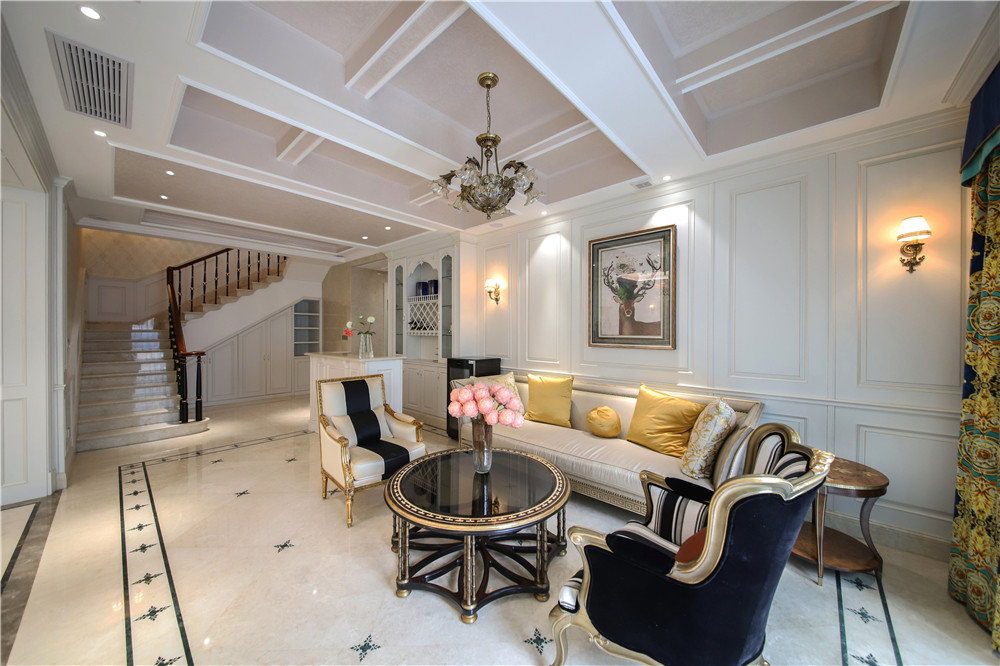负一层的空间配有休息区、酒吧品酒区、喝茶区、影音室、健身房、及保姆房等，各项功能极尽完善。与一层主客厅的充盈华贵不同，负一层休息区设计的清新脱俗，而更加注重功能性。淡雅的白色大理石地板和墙面使负一层的空间也丝毫不显黯然无光，反而为业主打造了一个优雅、自在、舒适的休闲空间。

茶室Tea room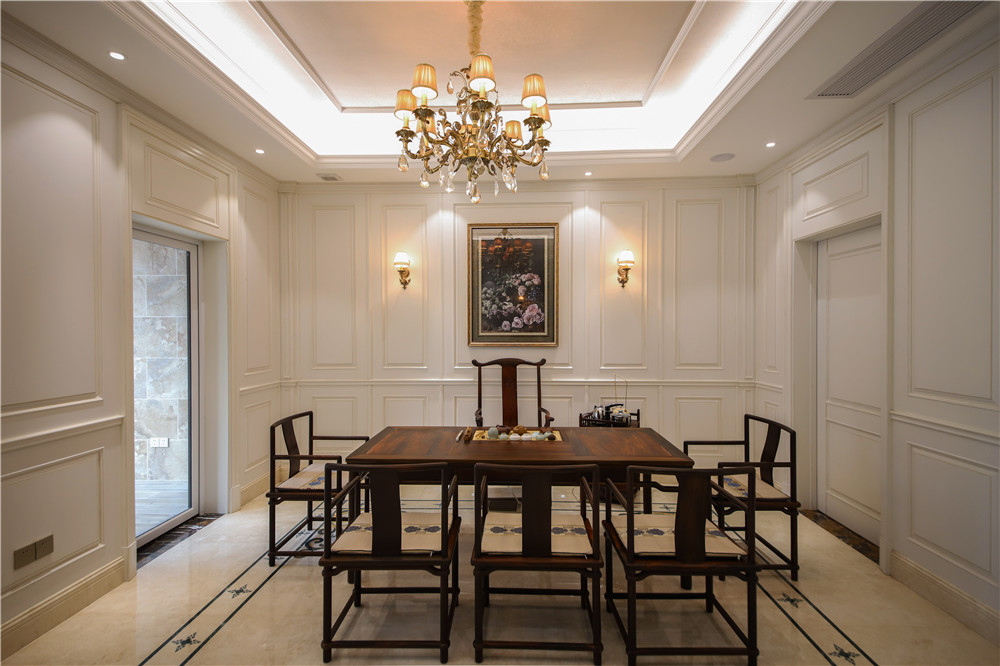同时在地下一层的品茶区的设计完美的融合了中西方风格，简单对称的墙体装饰搭配色调温馨的灯饰，用简约的凹凸层次和直线条很好的表现了低调奢华的欧式感觉；而中间放置的古色古香的深色木质桌椅，没有一丝多余的装饰，却将中式古典风展示的淋漓尽致，毫无违和感。

楼梯Stairs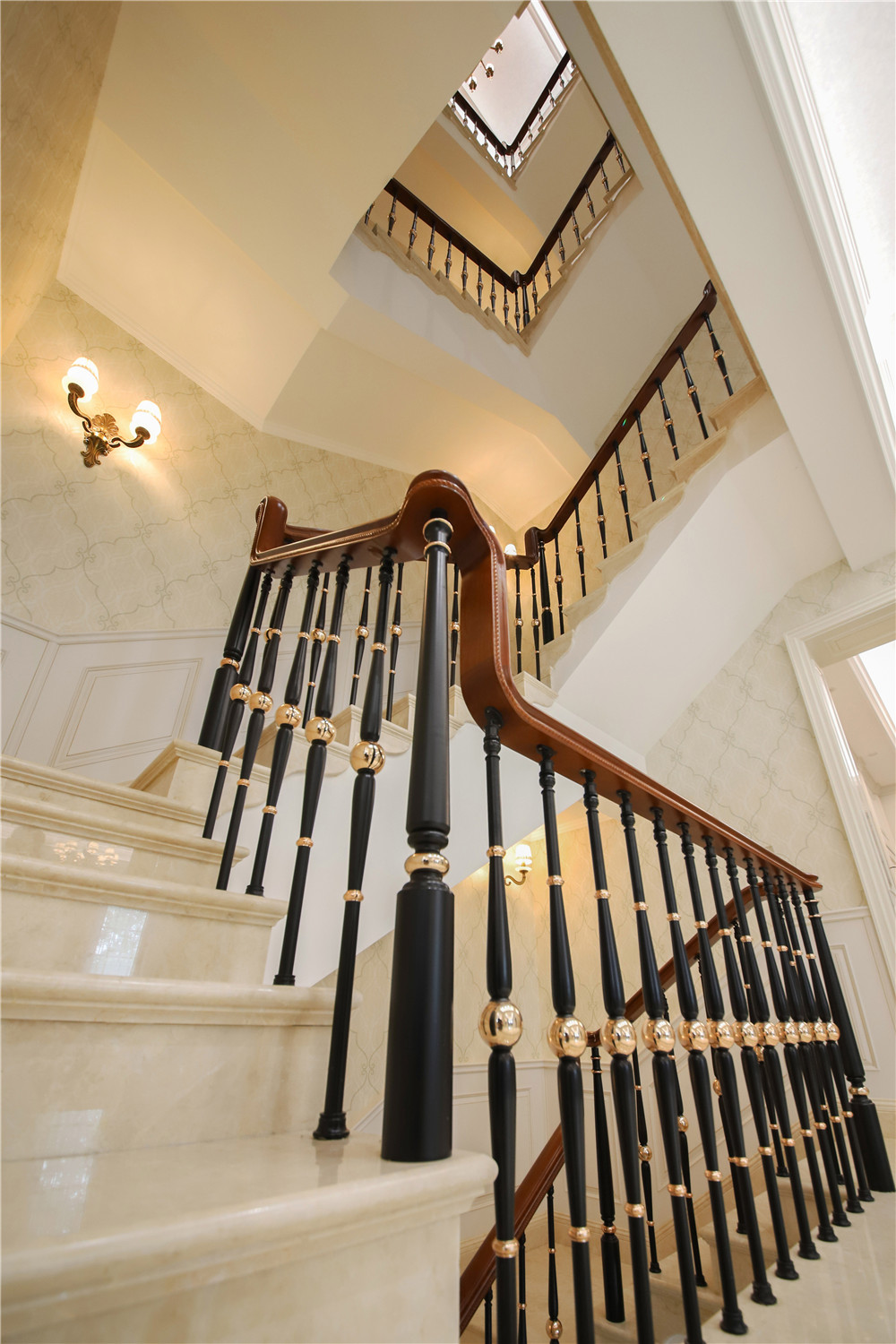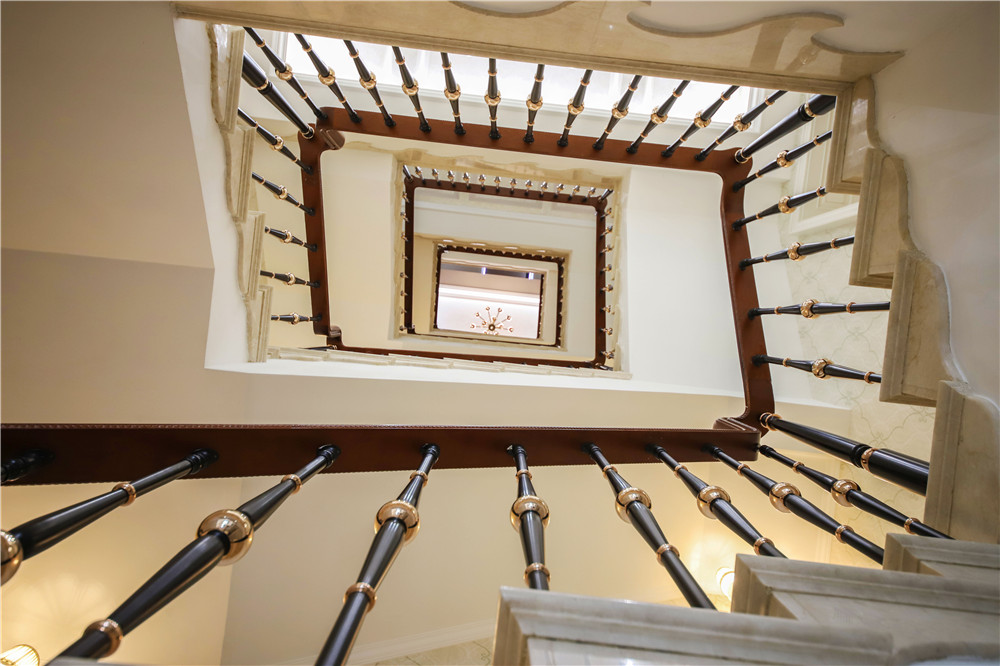设计师王乐乐常说，好的设计往往体现在每一个细节中。就好像本案中的楼梯设计，看似简单大气，但其实细节工艺考究。磨砂黑与金色相间的栏杆搭配深桃木色扶手，使得整个楼梯看上去极具质感，加上乳白色大理石台阶，让业主每走一步都能感受到安全和品质。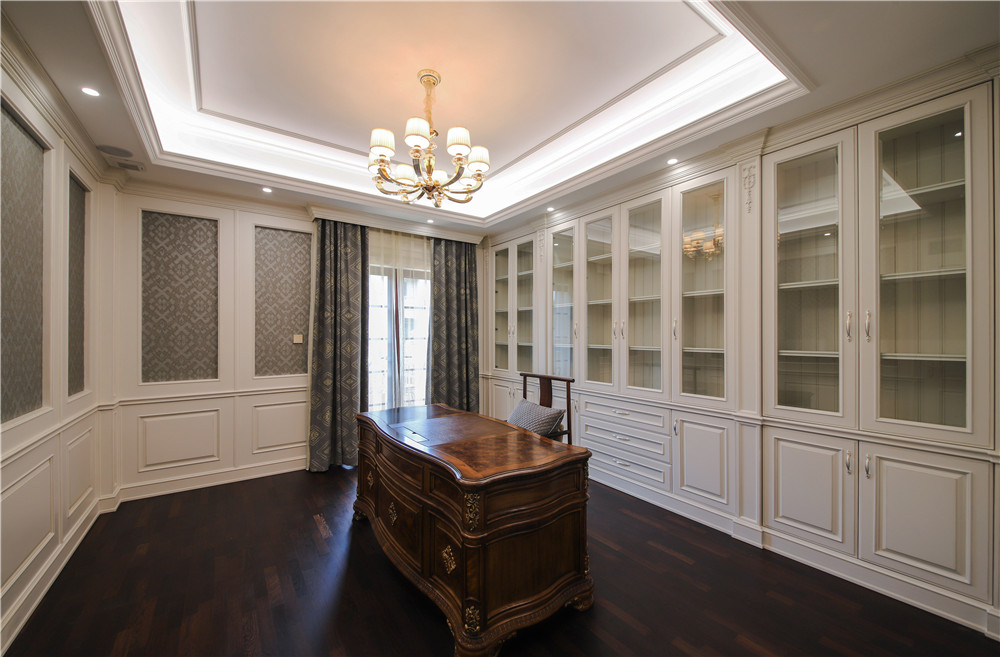顺梯而上，便到了家人的私密空间区。位于二楼的书房，装饰与茶室如出一辙，同样的干净利落，同样的清爽明目。进入书房，首先映入眼帘的是一整排白色书柜，在颜色与风格的选择上与整体的欧式风格融合的恰如其分，既充当了房间装饰，又增添了储物空间，不仅可以放书，还可以放置一些从世界各地带回来的精巧物件。而书房的深色木地板与房间正中的木质书桌为书房增添了沉稳的感觉，让人一坐下便能沉下心来，静品书香。

女孩房Girls 'room.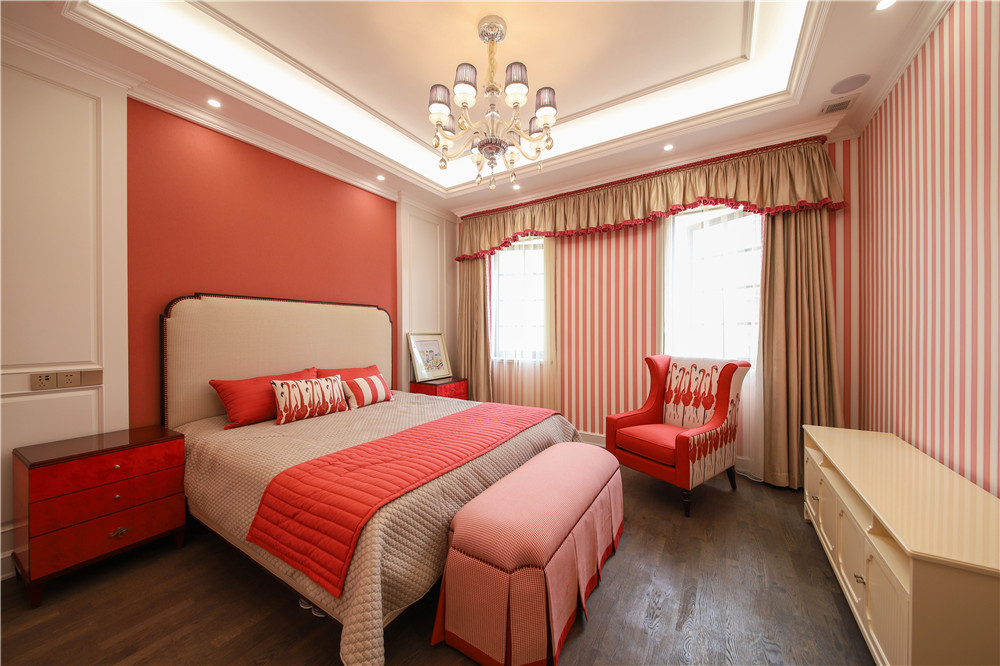走进女孩房，好像是走进了童话世界里的公主城堡，又好像是走进了粉红色的梦幻花园，但更像是走进了一个妙龄少女甜甜的笑眼里。踏入房间的第一步，就被眼前的色彩所吸引，粉红色的墙面、桃红色的装饰、香槟金的窗帘，到处都洋溢着少女的情怀和春天般馨甜的气息，让人流连忘返、不愿离去。

男孩房Boys 'room.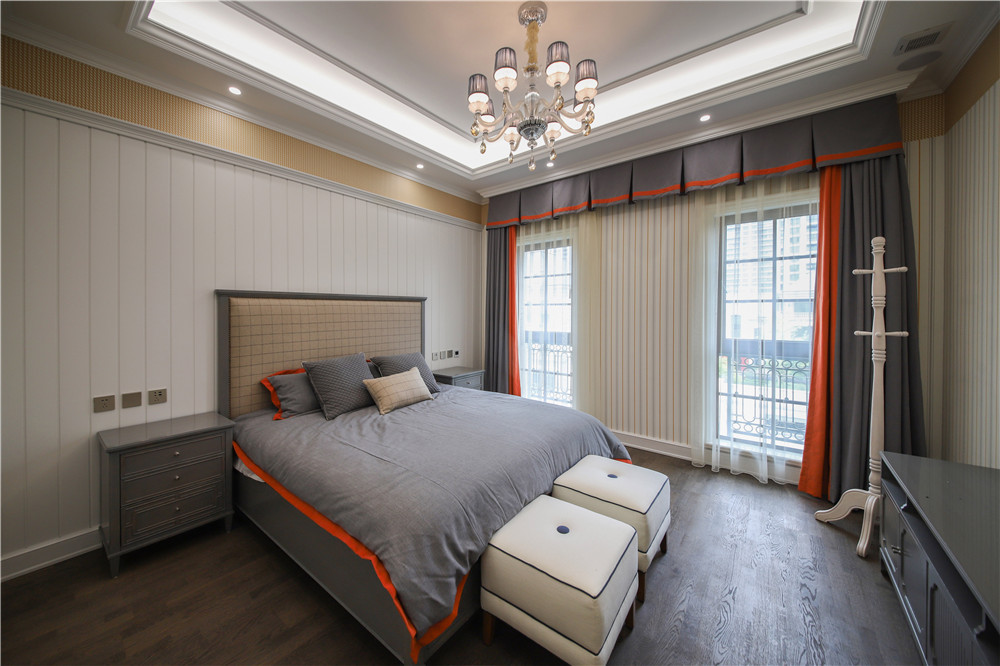位于三层的男孩房选用清爽活泼的蓝色调，点缀明艳动人的橘红色窗帘和床饰，干净清爽中又多了一份男孩儿的浪漫与天真。在空间设计上，设计师考虑到岁小男孩的日常生活所需，则没有进行过多繁杂的装饰，而是留有宽敞完备的空间，让孩子在这里尽情享受年轻生命的活力。

男孩卫生间Boy's bathroom.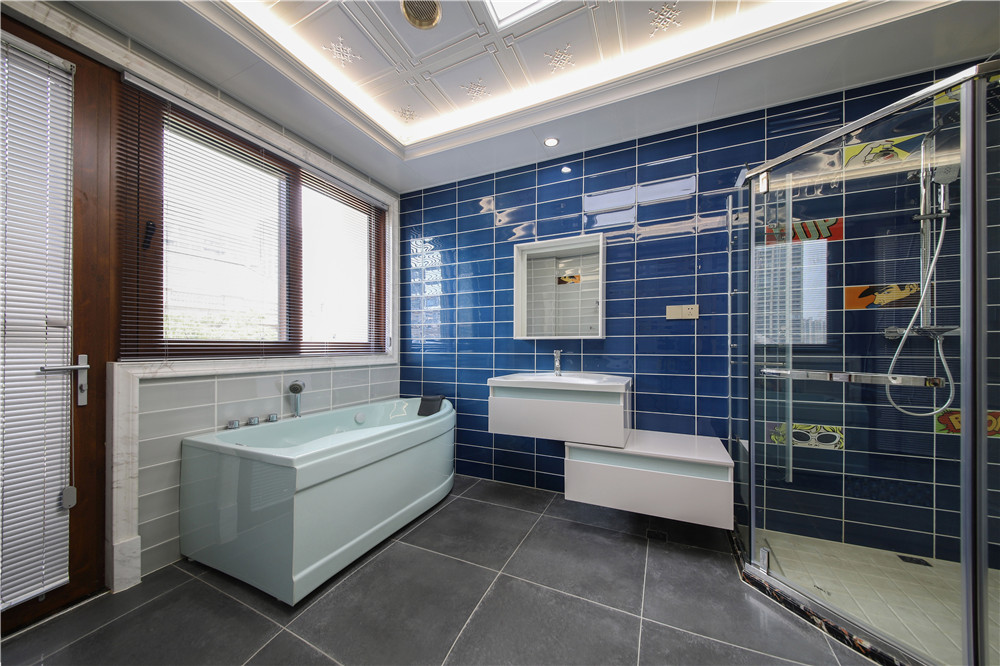男孩卫生间与男孩房的装饰风格一以贯之，同样选择灰色作为基调，但在颜色选择上更加大胆。深蓝色的墙面瓷砖不仅便于打理，更营造了种海洋的感觉。同时，设计师王乐乐在卫生间做了干湿分离处理，还专门为男孩设计了一个极具造型感的浴缸，大大增加了卫生间的美观性和实用性。

设计师介绍Designer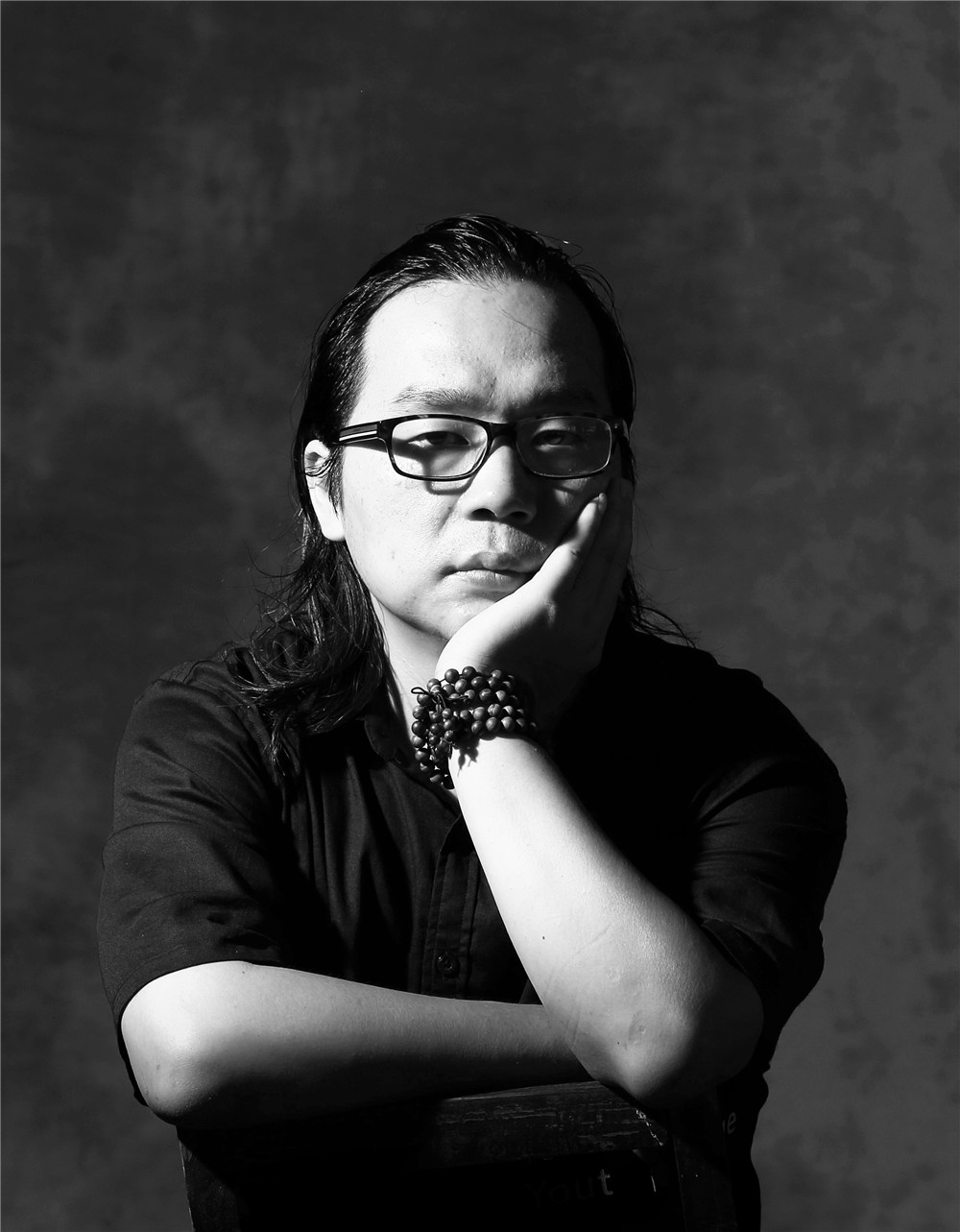设计师王乐乐

王乐乐高端室内设计工作室负责人兼创意总监

浙江皇嘉装饰有限公司创意总监

国家注册室内设计师

获得荣誉：

2005年代表上海全筑装饰设计公司荣获“日立杯”大赛银奖

2013年荣获北京国际设计周设计大赛“优秀奖”

2013年荣获度浙中地区装饰行业影响力设计师

2015年荣获“中国人生活方式”金华地区“城市十大设计师”称号

2016年荣获“艾特奖”最佳陈设艺术设计奖

2017年荣获 “艾特奖”最佳优秀设计奖

2018年荣获腾讯家居年度设计时尚盛典风采奖

主要作品：上海光明城市花园林公馆、上海魏玛别墅、上海玫瑰园73号别墅、 淀山湖会所等高档社区、上海太阳湖花园7号别墅、金华保集蓝郡别墅、金奥花园独幢别墅、缪斯酒吧、东阳冯德全国际双语幼儿园

`声明：本文由入驻焦点开放平台的作者撰写，除焦点官方账号外，观点仅代表作者本人，不代表焦点立场错误信息举报电话： 400-099-0099，邮箱：jubao@vip.sohu.com，或点此进行意见反馈，或点此进行举报投诉。`A B C D E F G H J K L M N P Q R S T W X Y Z
A - B - C - D - E
• A
• 鞍山
• 安庆
• 安阳
• 安顺
• 安康
• 澳门
• B
• 北京
• 保定
• 包头
• 巴彦淖尔
• 本溪
• 蚌埠
• 亳州
• 滨州
• 北海
• 百色
• 巴中
• 毕节
• 保山
• 宝鸡
• 白银
• 巴州
• C
• 承德
• 沧州
• 长治
• 赤峰
• 朝阳
• 长春
• 常州
• 滁州
• 池州
• 长沙
• 常德
• 郴州
• 潮州
• 崇左
• 重庆
• 成都
• 楚雄
• 昌都
• 慈溪
• 常熟
• D
• 大同
• 大连
• 丹东
• 大庆
• 东营
• 德州
• 东莞
• 德阳
• 达州
• 大理
• 德宏
• 定西
• 儋州
• 东平
• E
• 鄂尔多斯
• 鄂州
• 恩施
F - G - H - I - J
• F
• 抚顺
• 阜新
• 阜阳
• 福州
• 抚州
• 佛山
• 防城港
• G
• 赣州
• 广州
• 桂林
• 贵港
• 广元
• 广安
• 贵阳
• 固原
• H
• 邯郸
• 衡水
• 呼和浩特
• 呼伦贝尔
• 葫芦岛
• 哈尔滨
• 黑河
• 淮安
• 杭州
• 湖州
• 合肥
• 淮南
• 淮北
• 黄山
• 菏泽
• 鹤壁
• 黄石
• 黄冈
• 衡阳
• 怀化
• 惠州
• 河源
• 贺州
• 河池
• 海口
• 红河
• 汉中
• 海东
• 怀来
• I
• J
• 晋中
• 锦州
• 吉林
• 鸡西
• 佳木斯
• 嘉兴
• 金华
• 景德镇
• 九江
• 吉安
• 济南
• 济宁
• 焦作
• 荆门
• 荆州
• 江门
• 揭阳
• 金昌
• 酒泉
• 嘉峪关
K - L - M - N - P
• K
• 开封
• 昆明
• 昆山
• L
• 廊坊
• 临汾
• 辽阳
• 连云港
• 丽水
• 六安
• 龙岩
• 莱芜
• 临沂
• 聊城
• 洛阳
• 漯河
• 娄底
• 柳州
• 来宾
• 泸州
• 乐山
• 六盘水
• 丽江
• 临沧
• 拉萨
• 林芝
• 兰州
• 陇南
• M
• 牡丹江
• 马鞍山
• 茂名
• 梅州
• 绵阳
• 眉山
• N
• 南京
• 南通
• 宁波
• 南平
• 宁德
• 南昌
• 南阳
• 南宁
• 内江
• 南充
• P
• 盘锦
• 莆田
• 平顶山
• 濮阳
• 攀枝花
• 普洱
• 平凉
Q - R - S - T - W
• Q
• 秦皇岛
• 齐齐哈尔
• 衢州
• 泉州
• 青岛
• 清远
• 钦州
• 黔南
• 曲靖
• 庆阳
• R
• 日照
• 日喀则
• S
• 石家庄
• 沈阳
• 双鸭山
• 绥化
• 上海
• 苏州
• 宿迁
• 绍兴
• 宿州
• 三明
• 上饶
• 三门峡
• 商丘
• 十堰
• 随州
• 邵阳
• 韶关
• 深圳
• 汕头
• 汕尾
• 三亚
• 三沙
• 遂宁
• 山南
• 商洛
• 石嘴山
• T
• 天津
• 唐山
• 太原
• 通辽
• 铁岭
• 泰州
• 台州
• 铜陵
• 泰安
• 铜仁
• 铜川
• 天水
• 天门
• W
• 乌海
• 乌兰察布
• 无锡
• 温州
• 芜湖
• 潍坊
• 威海
• 武汉
• 梧州
• 渭南
• 武威
• 吴忠
• 乌鲁木齐
X - Y - Z
• X
• 邢台
• 徐州
• 宣城
• 厦门
• 新乡
• 许昌
• 信阳
• 襄阳
• 孝感
• 咸宁
• 湘潭
• 湘西
• 西双版纳
• 西安
• 咸阳
• 西宁
• 仙桃
• 西昌
• Y
• 运城
• 营口
• 盐城
• 扬州
• 鹰潭
• 宜春
• 烟台
• 宜昌
• 岳阳
• 益阳
• 永州
• 阳江
• 云浮
• 玉林
• 宜宾
• 雅安
• 玉溪
• 延安
• 榆林
• 银川
• Z
• 张家口
• 镇江
• 舟山
• 漳州
• 淄博
• 枣庄
• 郑州
• 周口
• 驻马店
• 株洲
• 张家界
• 珠海
• 湛江
• 肇庆
• 中山
• 自贡
• 资阳
• 遵义
• 昭通
• 张掖
• 中卫

1室1厅1厨1卫1阳台

1
2
3
4
5

0
1
2

1

1

0
1
2
3报名成功，资料已提交审核A B C D E F G H J K L M N P Q R S T W X Y Z
A - B - C - D - E
• A
• 鞍山
• 安庆
• 安阳
• 安顺
• 安康
• 澳门
• B
• 北京
• 保定
• 包头
• 巴彦淖尔
• 本溪
• 蚌埠
• 亳州
• 滨州
• 北海
• 百色
• 巴中
• 毕节
• 保山
• 宝鸡
• 白银
• 巴州
• C
• 承德
• 沧州
• 长治
• 赤峰
• 朝阳
• 长春
• 常州
• 滁州
• 池州
• 长沙
• 常德
• 郴州
• 潮州
• 崇左
• 重庆
• 成都
• 楚雄
• 昌都
• 慈溪
• 常熟
• D
• 大同
• 大连
• 丹东
• 大庆
• 东营
• 德州
• 东莞
• 德阳
• 达州
• 大理
• 德宏
• 定西
• 儋州
• 东平
• E
• 鄂尔多斯
• 鄂州
• 恩施
F - G - H - I - J
• F
• 抚顺
• 阜新
• 阜阳
• 福州
• 抚州
• 佛山
• 防城港
• G
• 赣州
• 广州
• 桂林
• 贵港
• 广元
• 广安
• 贵阳
• 固原
• H
• 邯郸
• 衡水
• 呼和浩特
• 呼伦贝尔
• 葫芦岛
• 哈尔滨
• 黑河
• 淮安
• 杭州
• 湖州
• 合肥
• 淮南
• 淮北
• 黄山
• 菏泽
• 鹤壁
• 黄石
• 黄冈
• 衡阳
• 怀化
• 惠州
• 河源
• 贺州
• 河池
• 海口
• 红河
• 汉中
• 海东
• 怀来
• I
• J
• 晋中
• 锦州
• 吉林
• 鸡西
• 佳木斯
• 嘉兴
• 金华
• 景德镇
• 九江
• 吉安
• 济南
• 济宁
• 焦作
• 荆门
• 荆州
• 江门
• 揭阳
• 金昌
• 酒泉
• 嘉峪关
K - L - M - N - P
• K
• 开封
• 昆明
• 昆山
• L
• 廊坊
• 临汾
• 辽阳
• 连云港
• 丽水
• 六安
• 龙岩
• 莱芜
• 临沂
• 聊城
• 洛阳
• 漯河
• 娄底
• 柳州
• 来宾
• 泸州
• 乐山
• 六盘水
• 丽江
• 临沧
• 拉萨
• 林芝
• 兰州
• 陇南
• M
• 牡丹江
• 马鞍山
• 茂名
• 梅州
• 绵阳
• 眉山
• N
• 南京
• 南通
• 宁波
• 南平
• 宁德
• 南昌
• 南阳
• 南宁
• 内江
• 南充
• P
• 盘锦
• 莆田
• 平顶山
• 濮阳
• 攀枝花
• 普洱
• 平凉
Q - R - S - T - W
• Q
• 秦皇岛
• 齐齐哈尔
• 衢州
• 泉州
• 青岛
• 清远
• 钦州
• 黔南
• 曲靖
• 庆阳
• R
• 日照
• 日喀则
• S
• 石家庄
• 沈阳
• 双鸭山
• 绥化
• 上海
• 苏州
• 宿迁
• 绍兴
• 宿州
• 三明
• 上饶
• 三门峡
• 商丘
• 十堰
• 随州
• 邵阳
• 韶关
• 深圳
• 汕头
• 汕尾
• 三亚
• 三沙
• 遂宁
• 山南
• 商洛
• 石嘴山
• T
• 天津
• 唐山
• 太原
• 通辽
• 铁岭
• 泰州
• 台州
• 铜陵
• 泰安
• 铜仁
• 铜川
• 天水
• 天门
• W
• 乌海
• 乌兰察布
• 无锡
• 温州
• 芜湖
• 潍坊
• 威海
• 武汉
• 梧州
• 渭南
• 武威
• 吴忠
• 乌鲁木齐
X - Y - Z
• X
• 邢台
• 徐州
• 宣城
• 厦门
• 新乡
• 许昌
• 信阳
• 襄阳
• 孝感
• 咸宁
• 湘潭
• 湘西
• 西双版纳
• 西安
• 咸阳
• 西宁
• 仙桃
• 西昌
• Y
• 运城
• 营口
• 盐城
• 扬州
• 鹰潭
• 宜春
• 烟台
• 宜昌
• 岳阳
• 益阳
• 永州
• 阳江
• 云浮
• 玉林
• 宜宾
• 雅安
• 玉溪
• 延安
• 榆林
• 银川
• Z
• 张家口
• 镇江
• 舟山
• 漳州
• 淄博
• 枣庄
• 郑州
• 周口
• 驻马店
• 株洲
• 张家界
• 珠海
• 湛江
• 肇庆
• 中山
• 自贡
• 资阳
• 遵义
• 昭通
• 张掖
• 中卫• 手机• 分享
• 设计
免费设计
• 计算器
装修计算器
• 入驻
合作入驻
• 联系
联系我们
• 置顶
返回顶部